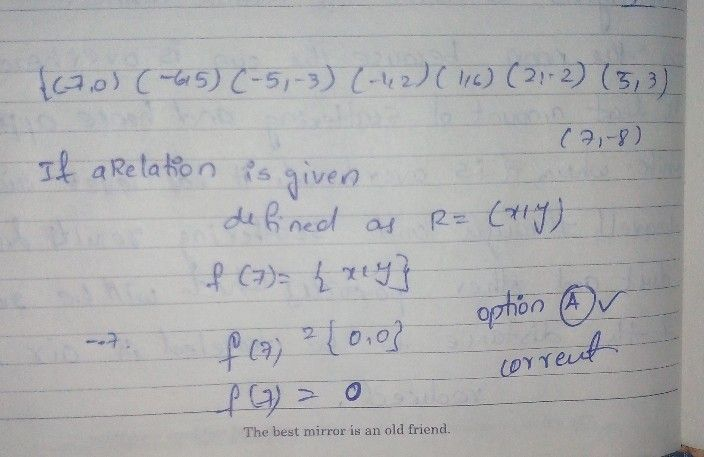Symbol
ProblemGiven the set of ordered pairs $\left(\left(-7.0\right),\left(-6,5\right),\left(-5,-3\right),\left(-1,2\right)$ $\left(1,6\right),\left(2,-2\right)$ $\left(5,3\right)\left(7,-8\right)\right)$ Find f(7)fAleft(7\right) O a O b -8 6. $5$